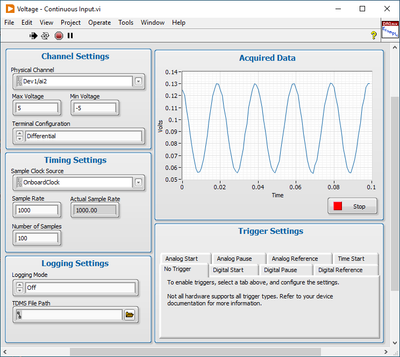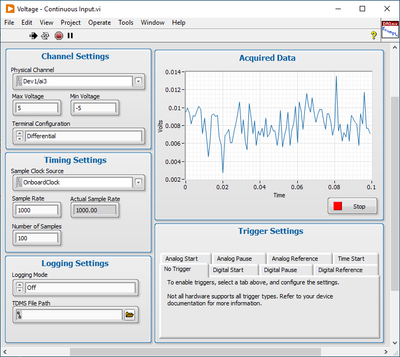# Multifunction DAQ

cancel
Showing results for
Did you mean:

## Getting incorrect voltage measurements overt resistor divider bridge

I have a problem where I need to measure power on three separate lanes with different power supplies (12V, 5V and 3,3V), however, my PCIe DAQ can only measure voltages up to 10V. To get good measurements I need to know the exact voltage on all lanes as well as the voltage drop over some shunt resistors. As advised in: DAQ change input voltage range I have built up the following resistor divider bridges:I am measuring the voltage in single ended ground referenced mode and the voltage drops over the shunts in differential mode. Problem is that the 5V and 3,3V seem to provide sensible readings while the 12V is inaccurate. I am expecting voltage drops in the order of 0,01V and when I measure with finite samples I get a sinusoidal with a mean value of 0,1V so 10 times the expected. If I change to continuous mode it becomes even worse with a measured voltage drop of about 0,5V so 50 times the expected. If I change both ai5+ and ai5- to single ended ground referenced and subtract them in software I seem to get a reasonable value but I am unsure if it is actually correct since I don't know what causes the problem. Can anybody give me an insight?

Message 1 of 28
(932 Views)

## Re: Getting incorrect voltage measurements overt resistor divider bridge

@Károly wrote:

I have a problem where I need to measure power on three separate lanes with different power supplies (12V, 5V and 3,3V), however, my PCIe DAQ can only measure voltages up to 10V. To get good measurements I need to know the exact voltage on all lanes as well as the voltage drop over some shunt resistors. As advised in: DAQ change input voltage range I have built up the following resistor divider bridges:I am measuring the voltage in single ended ground referenced mode and the voltage drops over the shunts in differential mode. Problem is that the 5V and 3,3V seem to provide sensible readings while the 12V is inaccurate. I am expecting voltage drops in the order of 0,01V and when I measure with finite samples I get a sinusoidal with a mean value of 0,1V so 10 times the expected. If I change to continuous mode it becomes even worse with a measured voltage drop of about 0,5V so 50 times the expected. If I change both ai5+ and ai5- to single ended ground referenced and subtract them in software I seem to get a reasonable value but I am unsure if it is actually correct since I don't know what causes the problem. Can anybody give me an insight?

Is "1m" really 1 milliohm? Or did you mean "1M" - 1 megaohm?

Message 2 of 28
(887 Views)

## Re: Getting incorrect voltage measurements overt resistor divider bridge

Rememba Ohm’s law and voltage divider formula and try to apply these formulas in your code, but it would be nice to see your code as well.

Message 3 of 28
(885 Views)

## Re: Getting incorrect voltage measurements overt resistor divider bridge

This seems like a pure hardware question, nothing to do with LabVIEW programming. See if you can find a more suitable board and I can move the tread for you.LabVIEW Champion.Message 4 of 28
(876 Views)

## Re: Getting incorrect voltage measurements overt resistor divider bridge

Yes, you are correct: 1m is 1 milliohm (small current shunt) and 10M is 10 Megaohm.

Message 5 of 28
(826 Views)

## Re: Getting incorrect voltage measurements overt resistor divider bridge

I am not calculating power yet. At this stage I'm only trying to measure correct voltage rails and correct voltage drops over the current shunts.

Here's the problem:On the left is the voltage drop over the 12V shunt and on the right is the 5V one. They both should be roughly 0,01V with some noise, however the 12V one is a sinusoidal with an incorrect amplitude.Here you can see the relevant code for the continuous measurement. Again the drop over the 5V shunt is reported correctly to be roughly 0,01V while the drop over the 12V shunt is incorrectly measured as 0,5V.

Message 6 of 28
(821 Views)

## Re: Getting incorrect voltage measurements overt resistor divider bridge

You haven't mentioned what exact DAQ card this is supposed to be. I'm particularly "surprised"  by the fact that the first 4 analog inputs seem to be single ended while the remaining 3 (4) seem to allow for differential operation. Depending on the actual card the negative input of those (differential) channels, may or may not be a valid input when operating in ground referenced mode!

Only higher end cards will support mixed mode operation with some being differential and others being single ended.

Rolf Kalbermatter
DEMO, TU Delft
My BlogMessage 7 of 28
(819 Views)

## Re: Getting incorrect voltage measurements overt resistor divider bridge

Dear Rolf,

Thank you for your answer. I'll update the question with this detail. The card I am using is a PCIe-6353 Series X DAQ.

Message 8 of 28
(815 Views)

## Re: Getting incorrect voltage measurements overt resistor divider bridge

I still insist that this thread is in the wrong place. Nothing to do with LabVIEW programming.

Should I move it to Multifunction DAQ or Signal Conditioning? (... or elsewhere).LabVIEW Champion.Message 9 of 28
(809 Views)

## Re: Getting incorrect voltage measurements overt resistor divider bridge

Not LabVIEW related however:

First thing: Take a look at the spec of your DAQ

https://www.ni.com/docs/de-DE/bundle/pcie-usb-6353-specs/page/specs.html

Fig 1 show the settle time error of this multiplexed card.  What value was your source impedance ?  (even 1M is not shown in this double log diagram :D)

Way out: Use buffer amplifiers  for the analog voltage readings and use highside current monitoring instrumentation amplifiers for your shunts. Reduce the 10M to 500k  for the voltage reading . If you want to read faster than DC check for impedance compensation.

EDIT: If possible move the shunts to GND, but still use 2 wire true diffential reading. Every wire is a resistor, every current in a resistor leads to a voltage! if you now use 1k resistors as voltage devider and choose a slow sample rate, you migth get some not too wrong results without active amplifiers.

And finally try to avoid multiplexed input cards!   Nothing but trouble!

Greetings from Germany
Henrik

LV since v3.1

“ground” is a convenient fantasy

'˙˙˙˙uıɐƃɐ lɐıp puɐ °06 ǝuoɥd ɹnoʎ uɹnʇ ǝsɐǝld 'ʎɹɐuıƃɐɯı sı pǝlɐıp ǝʌɐɥ noʎ ɹǝqɯnu ǝɥʇ'

Message 10 of 28
(768 Views)